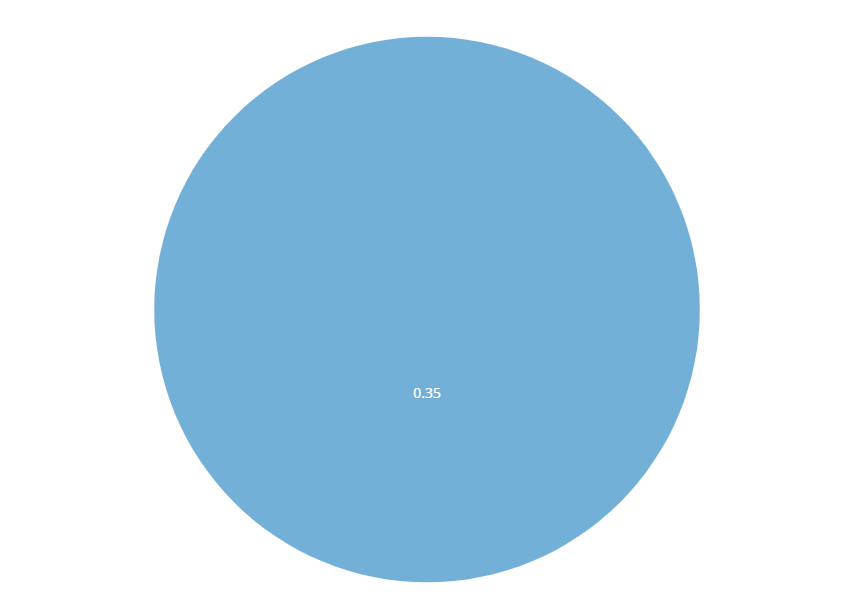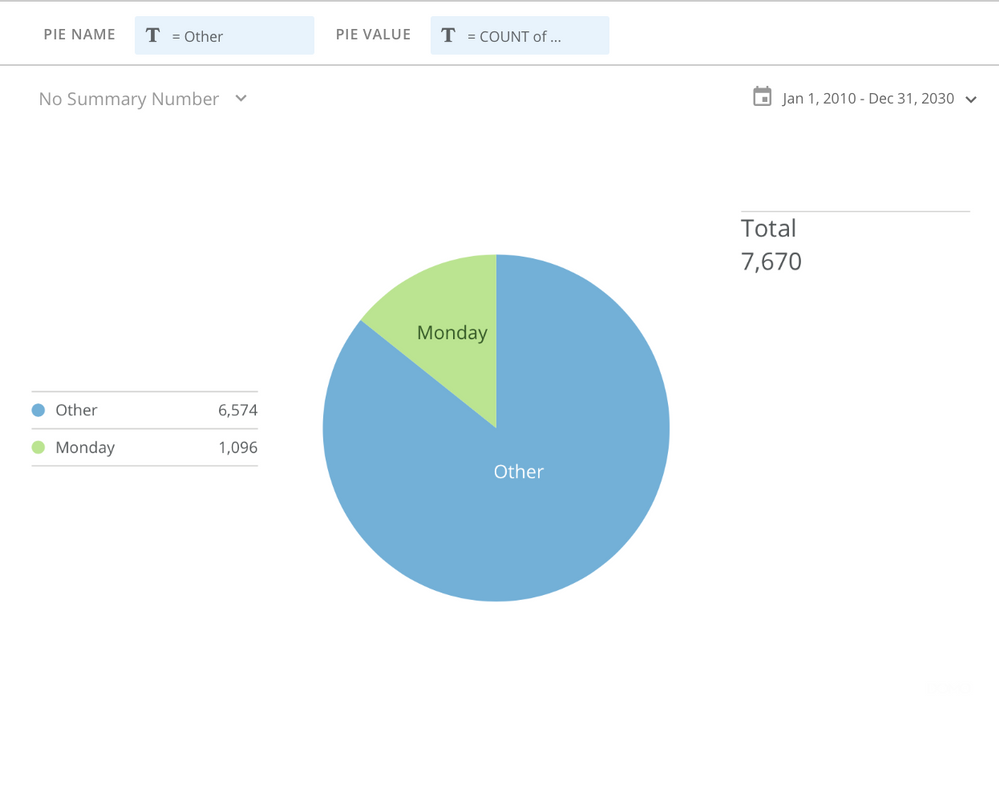# How to calculate other percentage in pie charts?

I have to create a pie chart in which one field calculation is like this

Sum(X) / Sum(Y) * 100

which is giving the value of a particular category ( M  ).

Now I have to show other categories percentages like 100 -  ( % of M ).

How can I do this in beast mode or without beast mode using pie charts?

How can I show  0.65 in other categories?•CoachWhat are your X and Y? Is Y constant across the entire dataset?

You could utilize a new beastmode to define a new category with a CASE statement and then use that as the Pie Name field

`CASE WHEN `dayName` = 'Monday' THEN `dayName`ELSE 'Other'END`**Did this solve your problem? Accept it as a solution!**
• X and Y both are numbers that vary by date range.

In your example, you used count here instead of counting I want some percentage calc

so my formula for Monday = Sum(X) / Sum(Y)

for Others 1 -  Sum(X) / Sum(Y)

So in this calc, I am getting the error.

If you have a solution for this scenario please share with your beast mode code.

•CoachThe Pie Chart automatically calculates the percentage based on the category label. Are you able to determine if a value is to be included in your X bucket or not? If so you can use that logic in a case statement to split out the X values into 'Other' and 'Not Other' buckets to graph how you're wanting.

Your math simplifies to SUM(NOT X) / SUM(Y) for the other grouping. So instead of thinking it like 1 - SUM(x) / SUM(Y) thinnk of it in terms of X or Not X for your grouping.

This is assuming your dataset isn't summarized via a SUM before utilizing it in the pie chart.

**Did this solve your problem? Accept it as a solution!**
• My Dataset can not be summarized via a SUM before utilizing it in the pie chart because the pie chart will show dynamic values based on date selection filter on the dashboard.

In my dataset, there are four categories ( A, B, C, D) out of two ( B and C ) ( I am creating a pie chart for these two. ) are having calculations like SUM(X) / SUM(Y) in a column but others have SUM(X) only.

This calculation  SUM(NOT X) / SUM(Y) and 1 - SUM(X) / SUM(Y) is wrong in my case.

Business logic is like that we have taken only sum(x) / sum(y) which is a constant value on a particular date range selection.

then I have to put the remaining part of the percentage ( 1 - SUM(X) / SUM(Y) ) in other categories.

I should not take Sum( not x ) in others' calculations it will give the wrong calculation.

so when I am doing the calculation for else part ( 1 - sum(x) / sum(y) ).

It is taking x of ( A and D ) which is wrong.

for others calculation should be like this 1 - Sum (X of B ) / Sum ( Y ) ( for pie chart of B )

•CoachDo you have some example data you can provide (that's either safe to share or make your own obfuscated dataset as an example)?

I'm still a little perplexed with how your data is structured and what exactly you're asking about.

If you're doing a pie chart for just B then are you filtering for only B data on your card?.

Is Y for ALL data across A, B, C and D? Just B? Just B and C?